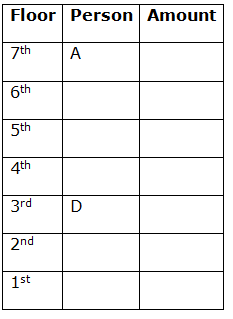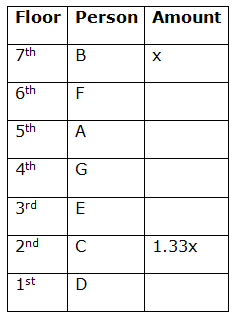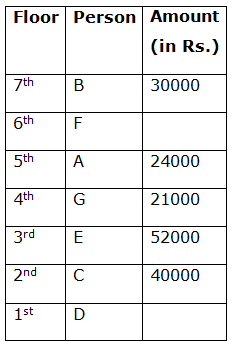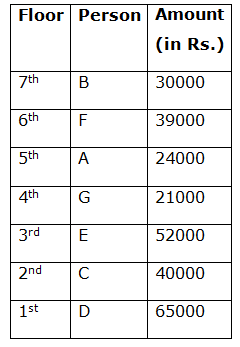# IBPS PO Mains Reasoning Questions 2019 – (Day-12)

Dear Aspirants, Our IBPS Guide team is providing new pattern Reasoning Questions for IBPS PO Mains 2019 so the aspirants can practice it on a daily basis. These questions are framed by our skilled experts after understanding your needs thoroughly. Aspirants can practice these new series questions daily to familiarize with the exact exam pattern and make your preparation effective.

Check here for IBPS PO Mains Mock Test 2019

[WpProQuiz 7432]

Puzzle

Direction (1-5): Study the following information carefully and answer the questions given below

Seven floors of a building were bought by seven different people, A, B, C, D, E, F, and G but not necessarily in the same order. After buying their flats, they spent some amount on the renovation of the flats. The amount spent by each person is different. The ground floor is numbered as the 1st floor, the floor above that is numbered 2nd floor and so on till the 7th floor.

B spent Rs. 10000 less than the person who lives on the 2nd floor but 6000 more than A. E lives just a floor above C, who spent 33.33% more than the person who is on the top floor. The amount spent by the person on the 6th floor is 60% of the amount spent by D, also the difference between their expenses is Rs. 26000. A lives four floors above D on an odd numbered floor. B lives three floors above G, who lives on a floor next to E’s floor. The person living on the 4th floor spent 12.5% less than the person living on a floor just above him but Rs. 31000 less than the person who lives just a floor below him.

1) Who lives three floors below the floor on which F lives?

a) E

b) G

c) C

d) A

e) D

2) What was the combined amount spent on renovation by the person who lives on the top and the bottom floor?

a) Rs. 82000

b) Rs. 95000

c) Rs. 87000

d) Rs. 78000

e) Rs. 83000

3) Who among the following bought the 6th floor?

A

B

G

F

E

4) What was the amount spent by the person on renovation who lives on the 3rd floor?

a) Rs. 20000

b) Rs. 39000

c) Rs. 52000

d) Rs. 65000

e) Rs. 35000

5) Who lives on the top floor?

E

F

D

G

None of the above

Computer aptitude

Directions (6-10): In a certain number system there are only two notations to represent numbers: @ and !.

0 is represented by @ and 1 by !. The subsequent numbers are represented in the following manner:

2 is represented as [email protected]

3 is represented as !!

4 is represented as [email protected]@

5 is represented as [email protected]! and so on.

Based on this coded language, answer the following questions.

6) Which of the following is not true?

b) !!! + [email protected]@@ = !!!!

e) None of them.

7) What is the cube root of half of [email protected]@@@@@@ ?

d) !!!

e) None of them.

8) Which is not a multiple of !!!?

c) [email protected]@@!!

e) None of them

9) What is the product of [email protected]! And !!! ?

a) [email protected]@@!!

c) [email protected]@@@!

d) [email protected]@@@@

e) None of them

10) What is the geometric mean of [email protected] and [email protected][email protected] ?

a) [email protected]@!!

b) [email protected]@@!

c) [email protected]@@@

e) None of them

Directions 1-5:

1) A lives four floors above D on an odd numbered floor.

(Implies A lives on either 5th floor or 7th floor and accordingly D lives on either 1st floor or 3rd floor respectively.)

2) E lives just a floor above C, who spent 33.33% more than the person who is on the top floor.

3) B lives three floors above G, who lives on a floor next to E’s floor.

(According to statement 1, let us assume that A lives on the 7th floor and D live on the 3rd floor.

The arrangement would look like this:(Now, if we place E & C above D, then we won’t be able to place B three floors above G, who is a neighbor of E. So we have to place E and C below D and there is only way this is possible i.e. E is on the 2nd floor and C is on the 1st floor. But now we can’t place G on a floor that is next to E’s floor because D and C are there already on the next floor.

Hence our assumption was wrong, A is on the 5th floor and D is on the 1st floor.

Now, it’s clear from statement 2 and 3 that G lives a floor above E (as C lives a floor below E) and B lives four floors above E.

So we cannot place E on the 4th floor, implies E lives on the 3rd floor, C lives a floor below him and G lives a floor above him and B lives on the top floor.

Let the amount spent by B (who lives on the top floor) = x

Then, the amount spent by C = 1.33x

Also, now we have the only person left to be mapped i.e. F, so we can place him on the 6th floor.)4) B spent Rs. 10000 less than the person who lives on the 2nd floor but 6000 more than A.

(Implies,

1.33x – x = Rs. 10000

x = Rs. 30000 = Amount spent by B

1.33x = Rs. 40000 = Amount spent by C

Amount spent by A = Rs. 30000 – Rs. 6000 = Rs. 24000)

5) The person living on the 4th floor spent 12.5% less than the person living on a floor just above him but Rs. 31000 less than the person who lives just a floor below him.

(Implies, G spent 87.5% the amount spent by A (who lives just a floor above him.

Amount spent by G = 87.5% of Rs. 24000

Amount spent by G = Rs. 21000

Also, E lives a floor below him and he spent = Rs. 21000 + Rs. 31000 = Rs. 52000.)6) The amount spent by the person on the 6th floor is 60% of the amount spent by D, also the difference between their expenses is Rs. 26000.

(Let the amount spent by D = y

Then, the amount spent by F = 0.6y

Also, y – 0.6y = Rs. 26000

0.5y=26000

y=13000

y = Rs. 65000 = Amount spent by D

0.6y = Rs. 39000 = Amount spent by F)Directions (6-10) :

On inspecting options,

[email protected][email protected] → 1010 which is the binary equivalent of

= (0 × 20) + (1 × 21) + (0 × 22) + (1 × 23)

= 0 + 2 + 0 + 8

= 10

!! → 11 which is the binary equivalent of

= (1 × 20) + (1 × 21)

= 1 + 2

= 3

The difference is 10 – 3 = 7, but

[email protected] → 110 which is the binary equivalent of

(0 × 20) + (1 × 21) + (1 × 22)

=0+2+4

=6

Hence [email protected][email protected] – !! = [email protected] is false.

[email protected]@@@@@@ → 10000000 which is the binary equivalent of

(0 × 20) + (0 × 21) + (0 × 22) + (0 × 23) + (0 × 24) + (0 × 25) + (0 × 26) + (1 × 27)

0 + 0 + 0 + 0 + 0 + 0 + 0 + 128

= 128.

Half of 128 is 64 and the cube root of 64 is 4.

The binary equivalent of 4 is 100 → [email protected]@

[email protected]@ →100

(0 × 20) + (0 × 21) + (1 × 22)

=0+0+4

=4

Hence,[email protected]@ is the correct answer.

!!! →111 which is the binary equivalent of

(1 × 20) + (1 × 21) + (1 × 22)

=1+2+4

=7

[email protected]@! → 11001 which is the binary equivalent of

(1 × 20) + (0 × 21) + (0 × 22) + (1 × 23) + (1 × 24)

= 1 + 0 + 0 + 8 + 16

= 25 which is not a multiple of 7

Hence [email protected]@! Is the correct answer.

[email protected]! → 101 which is the binary equivalent of 5.

!!! → 111 which is the binary equivalent of

(1 × 20) + (1 × 21) + (1 × 22)

= 1 + 2 + 4

= 7.

So the product is 35.

The binary equivalent of 35 is 32 + 2 + 1 which will be 100011 → [email protected]@@!!

Hence, [email protected]@@!! Is the correct answer.

[email protected] → 110 which is the binary equivalent of

(0 × 20) + (1 × 21) + (1 × 22)

= 0 + 2 + 4

= 6

[email protected][email protected] → 110110 which is the binary equivalent of

(0 × 20) + (1 × 21) + (1 × 22) + (0 × 23) + (1 × 24) + (1 × 25)

= 0 + 2 + 4 + 0 + 16 + 32

= 54

The geometric mean of 6 and 54 is √(6 × 54) = 18.

The binary equivalent of 18 is 16 + 2 which will be 10010 → [email protected]@[email protected]

Hence, ‘[email protected]@[email protected]’ is the correct answer.